## Square Pyramid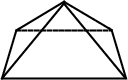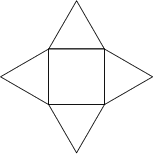A square pyramid is a Pyramid with a Square base. If the top of the pyramid is cut off by a Plane, a square Pyramidal Frustum is obtained. If the four Triangles of the square pyramid are Equilateral, the square pyramid is the regular'' Polyhedron known as Johnson Solid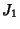and, for side length, has height(1)

Using the equation for a general Pyramid, the Volume of the regular'' is therefore(2)

If the apex of the pyramid does not lie atop the center of the base, then the Slant Height is given by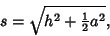(3)

whereis the height andis the length of a side of the base.Consider a Hemisphere placed on the base of a square pyramid (having side lengthsand height). Further, let the hemisphere be tangent to the four apex edges. Then what is the volume of the Hemisphere which is interior the pyramid (Cipra 1993)?

From Fig. (a), the Circumradius of the base is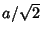. Now findin terms ofand. Fig. (b) shows a Cross-Section cut by the plane through the pyramid's apex, one of the base's vertices, and the base center. This figure gives(4)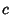(5)

so the Slant Height is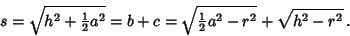(6)

Solving forgives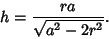(7)

We know, however, that the Hemisphere must be tangent to the sides, so, and(8)

Fig. (c) shows a Cross-Section through the center, apex, and midpoints of opposite sides. The Pythagorean Theorem once again gives(9)

We now need to findand.(10)

But we knowand, andis given by(11)

so(12)

Solving gives(13)

so(14)

We can now find the Area of the Spherical Cap as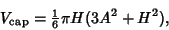(15)

where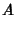(16)(17)

so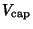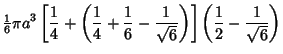(18)

Therefore, the volume within the pyramid is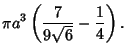(19)

This problem appeared in the Japanese scholastic aptitude test (Cipra 1993).

Cipra, B. An Awesome Look at Japan Math SAT.'' Science 259, 22, 1993.